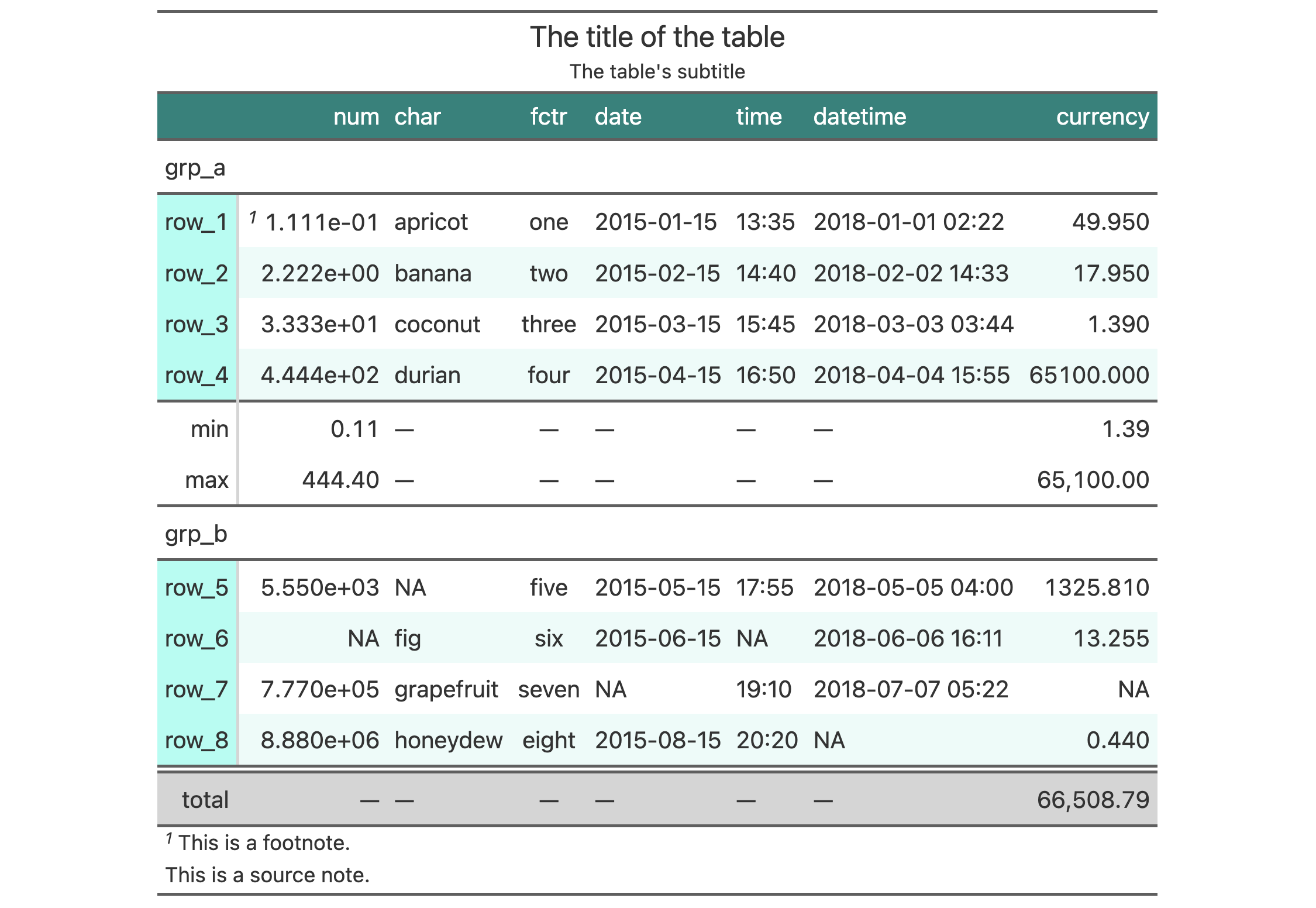With opt_stylize() you can quickly style your gt table with a carefully curated set of background colors, line colors, and line styles. There are six styles to choose from and they largely vary in the extent of coloring applied to different table locations. Some have table borders applied, some apply darker colors to the table stub and summary sections, and, some even have vertical lines. In addition to choosing a style preset, there are six color variations that each use a range of five color tints. Each of the color tints have been fine-tuned to maximize the contrast between text and its background. There are 36 combinations of style and color to choose from.

## Usage

opt_stylize(data, style = 1, color = "blue", add_row_striping = TRUE)

## Arguments

data

A table object that is created using the gt() function.

style

Six numbered styles are available. Simply provide a number from 1 (the default) to 6 to choose a distinct look.

color

There are six color variations: "blue" (the default), "cyan", "pink", "green", "red", and "gray".

An option to enable row striping in the table body for the style chosen. By default, this is TRUE.

## Value

an object of class gt_tbl.

## Examples

Use exibble to create a gt table with a number of table parts added. Then, use the opt_stylize() function to give the table some additional style (using the "cyan" color variation and style number 6).

exibble %>%
gt(rowname_col = "row", groupname_col = "group") %>%
summary_rows(
groups = "grp_a",
columns = c(num, currency),
fns = list(
min = ~min(., na.rm = TRUE),
max = ~max(., na.rm = TRUE)
)) %>%
grand_summary_rows(
columns = currency,
fns = list(
total = ~sum(., na.rm = TRUE)
)) %>%
tab_source_note(source_note = "This is a source note.") %>%
tab_footnote(
footnote = "This is a footnote.",
locations = cells_body(columns = 1, rows = 1)
) %>%
title = "The title of the table",
subtitle = "The table's subtitle"
) %>%
opt_stylize(style = 6, color = "cyan")## Function ID

9-10

Other table option functions: opt_align_table_header(), opt_all_caps(), opt_css(), opt_footnote_marks(), opt_horizontal_padding(), opt_row_striping(), opt_table_font(), opt_table_lines(), opt_table_outline(), opt_vertical_padding()